# color()

## Syntax

```color(mode=RGB, range=1.0) # the default color mode and range
color(range=255)           # use 0-255 component values rather than 0–1
color(HSV)                 # color-related commands will expect HSV values
```

Update the graphics state’s ‘current’ color mode

```color(r, g, b, a=1)    # RGB mode
color(h, s, v, a=1)    # HSV mode
color(c, m, y, k, a=1) # CMYK mode
```

Create a Color object using the current color mode

```color(v, a=1)
```

Create a greyscale Color object (regardless of the current mode)

## Color State

A number of plotdevice commands can take lists of numeric arguments to specify a color (see stroke(), fill(), background(), etc.). When called with keyword arguments alone, The color() command allows you to control how these numbers should be interpreted in subsequent color-modification commands.

By default, color commands interpret groups of 3 or more numbers as r/g/b triplets (with an optional, final alpha arg). If the `mode` keyword arg is set to `RGB`, `HSV`, or `CMYK`, subsequent color commands will interpret numerical arguments according to that colorspace instead.

The `range` keyword arg sets the maximum value for color channels. By default this is 1.0, but 255 and 100 are also sensible choices.

For instance here are three equivalent ways of setting the fill color to ‘blue’:

```color(mode=HSV)
fill(.666, 1.0, .76)
color(mode=RGB, range=255)
fill(0, 0, 190)
color(mode=CMYK, range=100)
fill(95, 89, 0, 0)
```

Color mode & range changes can be constrained to a block of code using the `with` statement. e.g.,

```background(.5, .5, .6) # interpteted as r/g/b (the default)
with color(mode=CMYK): # temporarily change the mode:
stroke(1, 0, 0, 0) # - interpteted as c/m/y/k
fill(1, 0, 0)          # outside the block, the mode is restored to r/g/b
```

## Making Colors

When called with a sequence of color-channel values, color() will return a reusable `Color` object. These can be passed to color-related commands in lieu of numeric args. For example:

```red = color(1, 0, 0)                   # r/g/b
glossy_black = color(15, 15, 15, 0.25) # r/g/b/a
background(red)
fill(glossy_black)
```

You can also prefix the numeric args with a color mode as a convenience for setting one-off colors in a mode different from the current colorspace:

```color(mode=HSV)                        # set mode to h/s/b
hsb_color = color(.7, 1, .8)           # uses current mode (h/s/b)
cmyk_color = color(CMYK, 0, .7, .9, 0) # interpreted as c/m/y/k
still_hsb = color(1, .5, .25)          # uses current mode (h/s/b)
```

Greyscale colors can be created regardless of the current mode by passing only one or two values (for the brightness and alpha respectively):

```fill(1, .75) # translucent white
stroke(0)    # opaque black
```

If you pass a string to a color command, it must either be a hex-string (beginning with a `#`) or a valid CSS color-name. The string can be followed by an optional alpha argument:

```background('#f00')   # blindingly red
fill('#74e9ff', 0.4) # translucent pale blue
stroke('chartreuse') # electric bile
```

## Returns

a Color object containing the defined color values

# fill()

## Syntax

```fill(r, g, b, a=1.0)
fill(h, s, v, a=1.0)
fill(c, m, y, k, a=1.0)
fill(v, a=1.0)
fill(color)
fill(*colors, angle, steps=[0,1]) # axial gradient
```

## Description

Sets the current fill color, interpeting the arguments according to the canvas’s current color-mode and range. For details see the color() command.

If more than one color arg is included, a gradient will be drawn. Pass a list with the `steps` keyword arg to set the relative location of each color in the gradient (0-1.0). The relative locations are based on the bounding-box of the object being filled (not its convex hull). So for highly rounded objects, you’ll need to adjust the steps to account for the dead-space in the corners of the bounds.

Including an `angle` keyword arg will draw a linear gradient at that orientation (otherwise it will be radial). Radial gradients will draw from the object center by default, but a relative center can be specified with the `center` keyword arg. If included, the center arg should be a 2-tuple with `x,y` values in the range -1 to +1.

In addition to colors, you can also call fill() with an image() as its sole argument. In this case the image will be tiled to fit the object being filled.

## Returns

a Color object representing the current fill color

## Example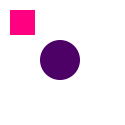```fill(1.0, 0.0, 0.5)
rect(10, 10, 25, 25)
fill(.3, 0.0, 0.4)
oval(40, 40, 40, 40)
```

# pen()

## Syntax

```pen(nib, join=MITER, cap=BUTT, dash=None)
```

## Description

Sets the line-style to be used when a stroke() color has been set. The `nib` argument sets the width of the stroke in terms of the units set in size() (points by default). The `cap` argument controls how endpoints of beziers are drawn: `BUTT`, `SQUARE`, or `ROUND`. Vertices within a bezier are controled by the `join` arg and can be set to `MITER`, `ROUND`, or `BEVEL`.

If the `dash` argument is not `None`, it must be either a single number or a list of them specifying on-off intervals for drawing a dashed line

## Returns

a context manager allowing pen changes to be constrained to the block of code following a `with` statement.

## Example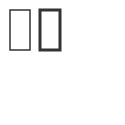```nofill()
stroke(0.2)
pen(1.5)
rect(10, 10, 20, 40)
pen(3)
rect(40, 10, 20, 40)
```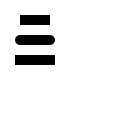```nofill()
stroke(0)
pen(10, cap=BUTT)
line(20,20, 50,20)
with pen(cap=ROUND):
line(20,40, 50,40)
with pen(cap=SQUARE):
line(20,60, 50,60)
```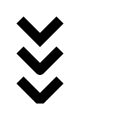```with nofill(), stroke(0), pen(10):
pen(join=MITER)
bezier([(20,20), (40,40), (60,20)])
pen(join=ROUND)
bezier([(20,50), (40,70), (60,50)])
pen(join=BEVEL)
bezier([(20,80), (40,100), (60,80)])
```

# stroke()

## Syntax

```stroke(r, g, b, a=1.0)
stroke(h, s, v, a=1.0)
stroke(c, m, y, k, a=1.0)
stroke(v, a=1.0)
stroke(color)
```

## Description

Sets the stroke color used when drawing lines around shapes, paths, and text. All drawing operations following a call to stroke() will be outlined in the specified color (with a ‘nib’ width set via the pen() command).

The color is specified in terms of `RGB`, `HSV` or `CMYK` depending on the canvas’s current color-mode and range. For details see the color() command. To disable stroke-drawing either call stroke() with `None` or use the nostroke() convenience method.

## Returns

a Color object representing the current stroke color

## Example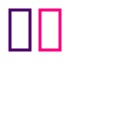```nofill()
strokewidth(3)
stroke(0.3, 0.0, 0.4)
rect(10, 10, 20, 40)
stroke(1.0, 0.0, 0.5)
rect(40, 10, 20, 40)
```

# Color

## Syntax

```Color()
```

## Description

The color commands in PlotDevice (see for example, color(), fill(), stroke(), background()) each return a Color object. This object has a number of properties to manipulate indivdual color values in different spaces and can be passed to the fill() and stroke() commands.

## Methods

```clr.blend(clr2, factor)
clr.copy()
```

The blend() method returns a new color that is a mixture of the object and another Color object. Set the `factor` argument to a value between 0 and 1.0 to control the proportions used for mixing the colors.

## Properties

```clr.r # .red
clr.g # .green
clr.b # .blue
clr.a # .alpha
```
```clr.h # .hue
clr.s # .saturation
clr.v # .value (or .brightness)
```
```clr.c # .cyan
clr.m # .magenta
clr.y # .yellow
clr.k # .black
```
```clr.hex  # a string like "#f00" or '#ff0000'
clr.hexa # a tuple like ("#aa99ff", .75)
```

## Syntax

```Gradient(*colors, steps=[0,1], angle=0)
```

```Gradient(*colors, steps=[0,1], center=[x,y])
```

## Description

A linear or radial gradient that can be used in any context that expects a Color (e.g., fill(), stroke(), background(), etc.). The `steps` list must have the same length as the number of colors and describes proportionally (in the range 0.0–1.0) where each color transition happens.

## Properties

`g.brightness`

## Methods

```g.fill(tuple(x, y, width, height))
g.fill(Bezier)```
```g.copy()
```

## Syntax

```Shadow(color, blur=10, offset=(5,5))
```

## Properties

```s.color
s.blur
s.offset
```

## Methods

```s.copy()
```

# capstyle()

## Syntax

```capstyle(style)
```

## Description

Defines how endpoints of a path should be rendered. The style argument must be one of: `BUTT` (the default), `ROUND`, or `SQUARE`. Note that the latter two styles extend the length of the path to add the endcaps.

## PlotDevice Equivalent

The pen() command allows you to set the linecap style using its `cap` argument.

## Example```fill(None)
stroke(0)
strokewidth(10)
capstyle(BUTT)
line(20,20, 50,20)
capstyle(ROUND)
line(20,40, 50,40)
capstyle(SQUARE)
line(20,60, 50,60)
```

# colormode()

## Syntax

```colormode(mode, range=1.0)
```

## Description

Changes the way PlotDevice interprets color values. By default, fill() and stroke() expect values between 0.0 and 1.0 using the RGB color model. `RGB` corresponds to Red/Green/Blue. Alternatively `HSV` (Hue/Saturation/Brightness) or `CMYK` (Cyan/Magenta/Yellow/Black) can be specified.

Regardless of the active colormode, PDFs and images are exported in the specified outputmode().

The optional range parameter specifies whether color values are defined between 0.0 and 1.0, or something else, 0 and 255 for example:

```colormode(range=255)
```

## Returns

the current color mode (`RGB`, `CMYK` or `HSV`)

## PlotDevice Equivalent

The mode used for interpreting color values is now set through the color() command. Color-related commands like fill(), stroke(), and background() can also take a mode as their first argument, specifying how the numerical args should be interpreted.

## Example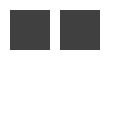```colormode(RGB)
fill(0.25, 0.25, 0.25)
rect(10, 10, 40, 40)

colormode(HSV)
fill(0, 0, 0.25)
rect(60, 10, 40, 40)
```

# joinstyle()

## Syntax

```joinstyle(style)
```

## Description

Defines how vertices in a path should be rendered. The style argument must be one of: `MITER` (the default), `ROUND`, or `BEVEL`.

## PlotDevice Equivalent

The pen() command allows you to set the linejoin style using its `join` argument.

## Example```fill(None)
stroke(0)
strokewidth(10)

joinstyle(MITER)
bezier([(20,20), (40,40), (60,20)])

joinstyle(ROUND)
bezier([(20,50), (40,70), (60,50)])

joinstyle(BEVEL)
bezier([(20,80), (40,100), (60,80)])
```

# nofill()

## Syntax

```nofill()
```

## Description

Disables the current fill color. All shapes, paths and text following this command will be drawn without a fill color. Calling nofill() is equivalent to `fill(None)`.

## Example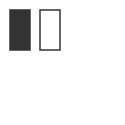```strokewidth(1.5)
stroke(0.2)
fill(0.2)
rect(10, 10, 20, 40)
nofill()
rect(40, 10, 20, 40)
```

# nostroke()

## Syntax

```nostroke()
```

## Description

Disables the current stroke color. All shapes, paths and text following this command will be drawn without a stroke (outline) color. Calling nostroke() is equivalent to `stroke(None)`.

## Example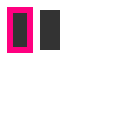```fill(0.2)
strokewidth(6)
stroke(1.0, 0.0, 0.5)
rect(10, 10, 20, 40)
nostroke()
rect(40, 10, 20, 40)
```

# strokewidth()

## Syntax

```strokewidth(width)
```

## Description

Sets the width of the stroke used for lines around shapes, paths and text.

## Returns

the current stroke width

## PlotDevice Equivalent

The pen() command allows you to set the stroke width using its `nib` keyword argument or simply by passing the new width as its first positional arg.

## Example```nofill()
stroke(0.2)
strokewidth(1.5)
rect(10, 10, 20, 40)
strokewidth(3)
rect(40, 10, 20, 40)
```